Question

Which of the following molecular geometries (shapes) are always Polar if 1 of the atoms around the central atom is...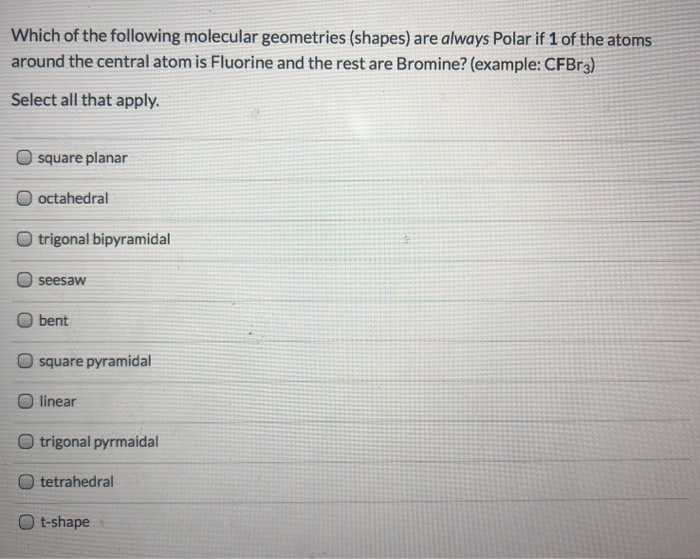Which of the following molecular geometries (shapes) are always Polar if 1 of the atoms around the central atom is Fluorine and the rest are Bromine? (example: CFBr3) Select all that apply. square planar O octahedral trigonal bipyramidal seesaw bent square pyramidal O linear trigonal pyrmaidal tetrahedral t-shape

first write the shapes and then based on that identify the polar or non polar as follows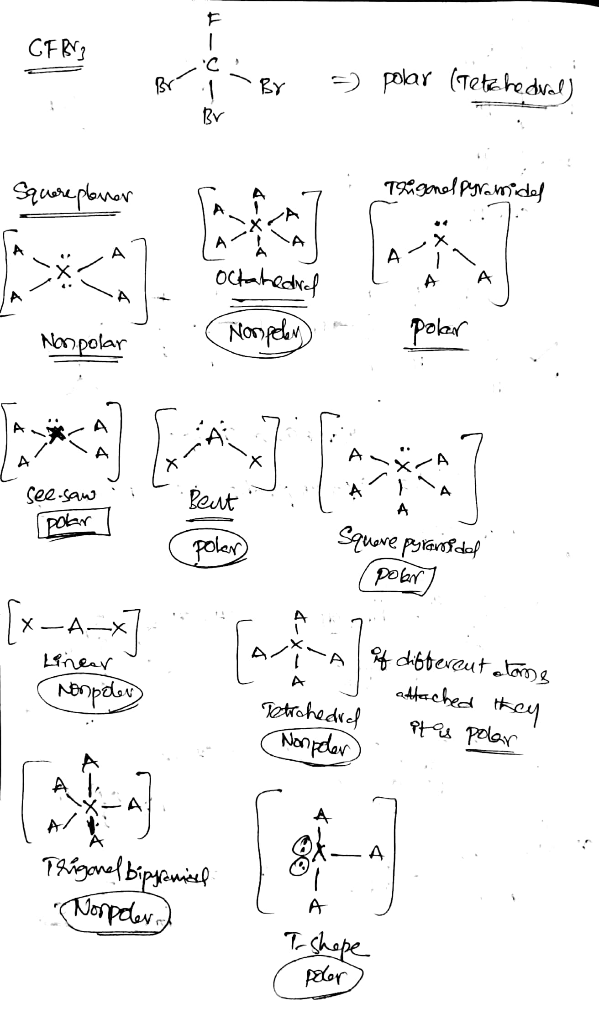Earn Coins

Coins can be redeemed for fabulous gifts.

Similar Homework Help Questions
• Which of the following molecular geometries (shapes) are always Polar if 2 of the atoms around...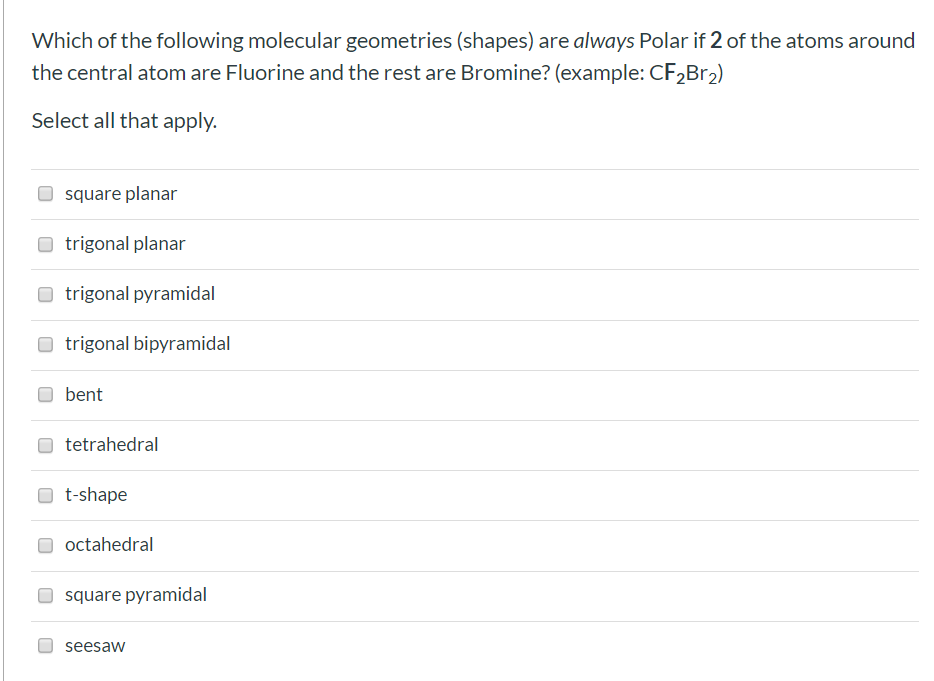Which of the following molecular geometries (shapes) are always Polar if 2 of the atoms around the central atom are Fluorine and the rest are Bromine? (example: CF2Br2) Select all that apply. square planar trigonal planar trigonal pyramidal trigonal bipyramidal bent tetrahedral t-shape octahedral square pyramidal seesaw

• Using VSEPR theory, a molecule with the general formula AX E# (A is the central atom,...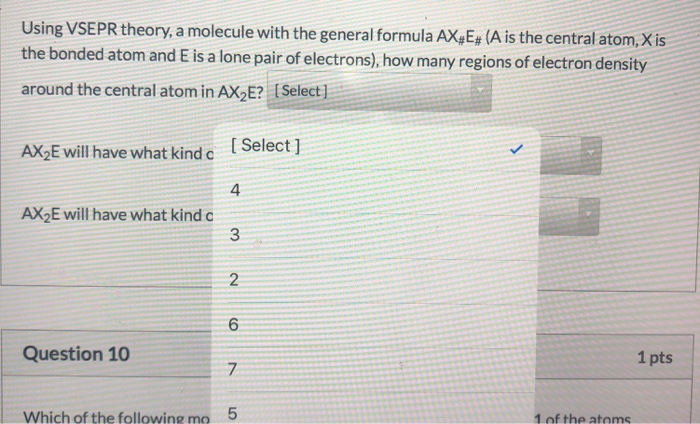Using VSEPR theory, a molecule with the general formula AX E# (A is the central atom, Xis the bonded atom and E is a lone pair of electrons), how many regions of electron density around the central atom in AX E? [Select] AX2E will have what kind c [Select] AX2E will have what kind a w N o Question 10 1 pts V Which of the following mo 5 1 of the atoms 5:35 PM Using VSEPR theory, a molecule...

• VSEPR theory predicts which shape for the molecular structure of the following molecules or molecular ions?...

VSEPR theory predicts which shape for the molecular structure of the following molecules or molecular ions? (i.e. the arrangement of only the bonded atoms about the central atom) bent (or angular) linear octahedral see-saw square planar square pyramidal tetrahedral trigonal bipyramidal trigonal planar trigonal pyramidal T-shaped  ICl3 molecule    (The central atom is I.) bent (or angular) linear octahedral see-saw square planar square pyramidal tetrahedral trigonal bipyramidal trigonal planar trigonal pyramidal T-shaped  IO4- ion    (The central atom is I.) bent (or angular) linear...

• Provide the correct molecular geometry for (c), given the number of lone pairs and bonding groups...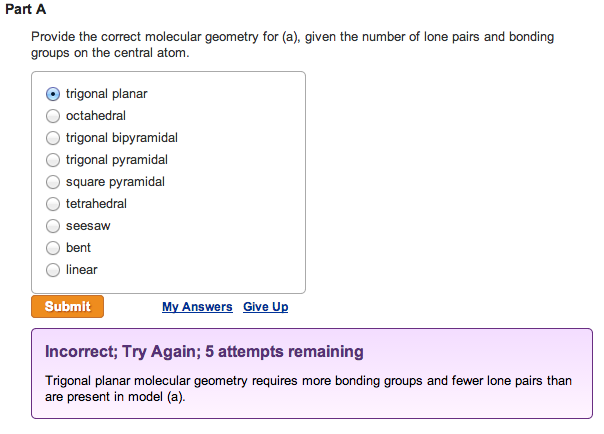Provide the correct molecular geometry for (a), given the number of lone pairs and bonding groups on the central atom. trigonal planar  octahedral  trigonal bipyramidal  trigonal pyramidal  square pyramidal  tetrahedral  seesaw  bent  linearProvide the correct molecular geometry for (b), given the number of lone pairs and bonding groups on the central atom.  trigonal bipyramidal  square pyramidal  bent  tetrahedral  trigonal planar  seesaw  trigonal pyramidal  octahedral  linear Provide the correct molecular geometry for (c), given the number of lone pairs and bonding groups on the...

• According to VSEPR theory, XeO3 has how many regions of electron density around the central atom...

According to VSEPR theory, XeO3 has how many regions of electron density around the central atom (how many electron groups?) (enter a number from 1 to 6) ? XeO3 will have (linear, trigonal planar, tetrahedral, trigonal pyramidal, bent, trigonal bipyramidal, seesaw, T-shaped, octahedral, square pyramidal, square planar) electronic geometry, and (linear, trigonal planar, tetrahedral, trigonal pyramidal, bent, trigonal bipyramidal, seesaw, T-shaped, octahedral, square pyramidal, square planar) molecular geometry. Draw the best Lewis structure using formal charge information. How many single...

• Please answer correctly I'll give best rating if you do correct one Electron Geomctry Name Choices:...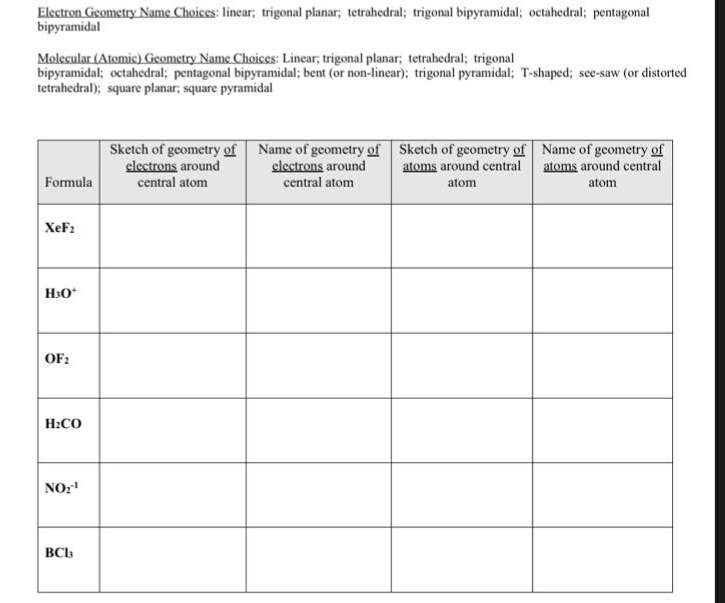Please answer correctly I'll give best rating if you do correct one Electron Geomctry Name Choices: linear; trigonal planar; tetrahedral; trigonal bipyramidal; octahedral; pentagonal bipyramidal Molecular (Atomic) Geometry Name Choices: Linear, trigonal planar; tetrahedral; trigonal bipyramidal; octahedral; pentagonal bipyramidal; bent (or non-linear); trigonal pyramidal: T-shaped; see-saw (or distorted tetrahedral); square planar, square pyramidal Sketch of geometry of Name of geometry of Sketch of geometry of Name of geometry of clectrons around central atom clectrons around atoms around central atoms around...

• Number 1-6 1. What is the molecular geometry of SBr4? A) seesaw B) square planar C)...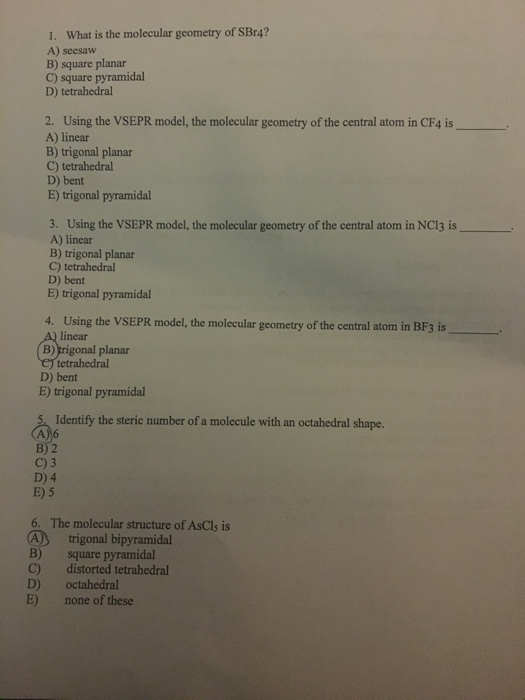Number 1-6 1. What is the molecular geometry of SBr4? A) seesaw B) square planar C) square pyramidal D) tetrahedral 2. Using the VSEPR model, the molecular geometry of the central atom in CF4 is A) linear B) trigonal planar C) tetrahedral D) bent E) trigonal pyramidal 3. Using the VSEPR model, the molecular geometry of the central atom in NC13 is A) linear B) trigonal planar C) tetrahedral D) bent E) trigonal pyramidal 4. Using the VSEPR model, the...

• Question 3 3.34 pts Determine the shape around each central atom in the acetate ion. Draw...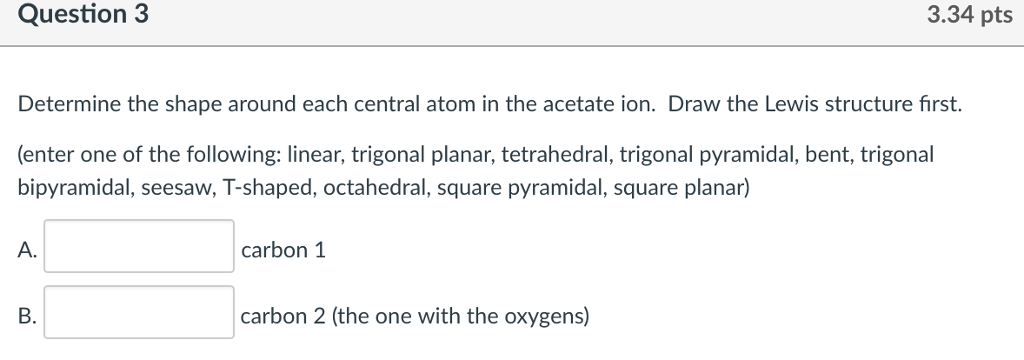Question 3 3.34 pts Determine the shape around each central atom in the acetate ion. Draw the Lewis structure first. trigonal pyramidal, bent. bipyramidal, seesaw, T-shaped, octahedral, square pyramidal, square planar) A. carbon 1 B. carbon 2 (the one with the oxygens)

• COORDINATION AND GEOMETRY TO2/S09 Use the abbreviations (table) to enter all possible geometries for the species...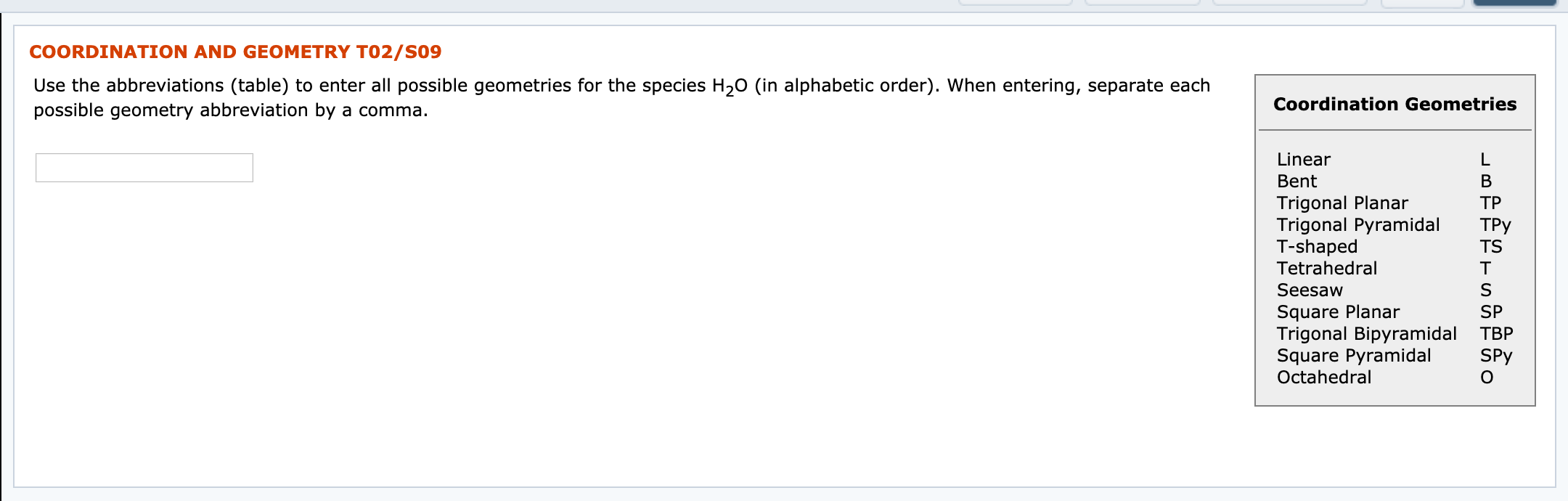COORDINATION AND GEOMETRY TO2/S09 Use the abbreviations (table) to enter all possible geometries for the species H20 (in alphabetic order). When entering, separate each possible geometry abbreviation by a comma. Coordination Geometries ТРу Linear Bent Trigonal Planar ТР Trigonal Pyramidal T-shaped TS Tetrahedral Seesaw Square Planar Trigonal Bipyramidal TBP Square Pyramidal Octahedral T SPY

• What are the VSEPR shapes (molecular geometries) associated with the indicated atoms I, II, III, respectively?!...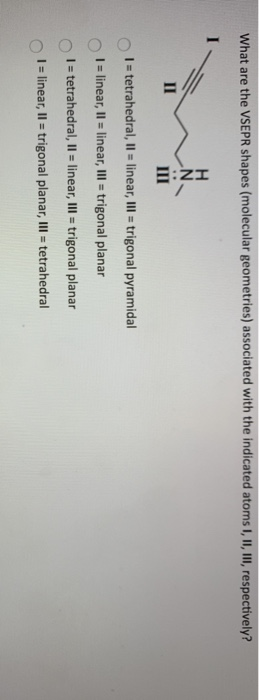What are the VSEPR shapes (molecular geometries) associated with the indicated atoms I, II, III, respectively?! N 1 = tetrahedral, II = linear, III = trigonal pyramidal T = linear, 1l = linear, III = trigonal planar 1 = tetrahedral, II = linear, III = trigonal planar I = linear, II = trigonal planar, III = tetrahedral